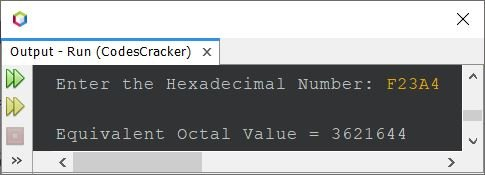# Java Program to Convert Hexadecimal to Octal

This post covers a program in Java that converts a given hexadecimal number to an octal number. You can refer to Hexadecimal to Octal, to understand the steps involved in the conversion.

## Hexadecimal to Octal Conversion in Java

The question is, write a Java program to convert hexadecimal number to octal. The hexadecimal number must be received by user at run-time of the program. Answer to this question, is the program given below:

```import java.util.Scanner;
import java.lang.Math;

public class CodesCracker
{
public static void main(String[] args)
{
int decimal=0, rem, i=0, len;
int[] octal = new int;

Scanner s = new Scanner(System.in);

len--;

while(len>=0)
{
if(rem>=48 && rem<=57)
rem = rem-48;
else if(rem>=65 && rem<=70)
rem = rem-55;
else if(rem>=97 && rem<=102)
rem = rem-87;
else
{
return;
}
decimal = (int) (decimal + (rem*Math.pow(16, i)));
i++;
len--;
}

i=0;
while(decimal!=0)
{
octal[i] = decimal%8;
i++;
decimal = decimal/8;
}

System.out.print("\nEquivalent Octal Value = ");
for(i=(i-1); i>=0; i--)
System.out.print(octal[i]);
}
}```

The sample run of above program with user input F23A4 as hexadecimal number to convert and print its equivalent octal value, is shown in the snapshot given below:The above program can also be created like the program given below. This program does not uses Math.pow() method. I've applied for loop in this program, instead of while. Also, this program uses directly the character to compare, instead of its ASCII value:

```import java.util.Scanner;

public class CodesCracker
{
public static void main(String[] args)
{
int decimal=0, rem, i=0;
int[] octal = new int;

Scanner s = new Scanner(System.in);

for(len=(len-1); len>=0; len--)
{
if(rem>='0' && rem<='9')
rem = rem-48;
else if(rem>='A' && rem<='F')
rem = rem-55;
else if(rem>='a' && rem<='f')
rem = rem-87;
else
{
return;
}
int m=1;
for(int k=1; k<=i; k++)
m *= 16;
decimal = (int) (decimal + (rem*m));
i++;
}

for(i=0; decimal!=0; decimal /= 8)
octal[i++] = decimal%8;

System.out.print("\nEquivalent Octal Value = ");
for(i=(i-1); i>=0; i--)
System.out.print(octal[i]);
}
}```

#### Same Program in Other Languages

Java Online Test

« Previous Program Next Program »